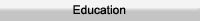Differential and Integral Equations.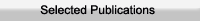1. A.Adawi & A.Alawneh (2003), A Parseval-type Theorem Applied to Certain Integral Transforms on Generalized Functions, IMA Journal of Applied Mathematics, Vol. 68, pages 587-593. 2. A.Adawi & F.Awawdeh (2008), New Approach to Compute Integral Transforms, Journal of Mathematics and Statistics, Vol. 4, No. 2, pages 94-97. 3. F.Awawdeh, A.Adawi & S.AlShara (2008), Analytic Solution of Multipantograph Equation , Journal of Applied Mathematics and Decision Sciences, Vol. 2008, Article ID 605064, 10 pages, doi:10.1155/2008/605064. 4. A.Adawi ,F.Awawdeh & H.Jaradat (2009), A Numerical Method for Solving Linear Integral Equations, International Journal of Contemporary Mathematical Sciences ,Vol.4, No10, pages 485-496. 5. F.Awawdeh, A.Adawi & S.AlShara (2009), A Numerical Method for Solving NonLinear Integral Equations , International Mathematical Forum ,Vol 4, No17, pages 805-817. 6.F.Awawdeh, A.Adawi & Z.Mustafa(2009),Solution of the SIR models of epidemics using HAM, Chaos , Solitons and Fractals,Vol 42 ,3047-3052. 7. A.Adawi(2010), Existence and Uniqueness Theorems of Higher Order Fractional Differential Equations. Journal of Applied Sciences, Vol 10, No. 18, pages 2132-2135. 8. M.Abu Shawiesh, J. Sinsomboonthong, A.Adawi,M. Almomani(2020),Confidence Intervals for the scale parameter of a two-parameter Weibull distribution: One sample problem. International Journal of Applied Mathematics,Vol 33 ,No 3 , pages 451-478.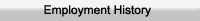1993-1995 The Private Zaytoonah University Jordan 1995-1998 The Hashemite University Jordan 1999- The Hashemite University Jordan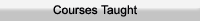Calculus, Mathematics for economics, Mathematics for Engineering, Differential Equations , Methods of Statistics, Linear Algebra, Euclidian Geometry , Vector Analysis, Special Functions, Probability Theory, Abstract Algebra, Complex Analysis, Topology, Integral Equations,Linear Programming, Number Theory.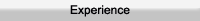1993-1995 The Private Zaytoonah University Jordan 1995-1998 The Hashemite University Jordan 1999- The Hashemite University Jordan